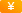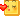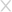## 从零编写一个自己的蜜罐系统

2019-03-06297051人围观 ，发现 4 个不明物体

*本文作者：lonely_wind，本文属 FreeBuf 原创奖励计划，未经许可禁止转载。

## 二、项目架构介绍

``````class RedisServer(Protocol):

def __init__(self):
pass

pass

self.transport.write()

def connectionLost(self, reason):
pass``````

2. 定义一个RedisServerFactory类，继承ServerFactory，用于创建RedisServer的实例

``````class RedisServerFactory(ServerFactory):
protocol = RedisServer``````

3. 使用reactor启动程序，并监听端口

``````reactor.listenTCP(6379, RedisServerFactory())
reactor.run()``````

## 三、协议格式解析

1. 简单字符串 Simple Strings, 以 “+”加号 开头

``格式：+ 字符串 \r\n``

``eg: "+OK\r\n"``

2. 错误 Errors, 以”-”减号 开头

``格式：- 错误前缀 错误信息 \r\n``

``eg:"-Error unknow command 'foobar'\r\n"``

3. 整数型 Integer， 以 “:” 冒号开头

``格式：: 数字 \r\n``

``eg:":1000\r\n"``

4. 大字符串类型 Bulk Strings, 以 “\$”美元符号开头，长度限制512M

``格式：\$ 字符串的长度 \r\n 字符串 \r\n``

``eg: "\$6\r\nfoobar\r\n" 其中字符串为foobar，而6就是foobar的字符长度``

5. 数组类型 Arrays，以 “*”星号开头

``格式：* 数组元素个数 \r\n 其他所有类型 ( 结尾不需要\r\n)``

``eg: "*0\r\n" 空数组``

``*3\r\n\$3\r\nset\r\n\$4\r\nname\r\n\$1\r\n1``

*3代表的是数组的长度为3（数据长度是以空格作为分隔符统计），\r\n是回车换行,

\$n代表每个字符串的长度，后面是字符串。## 四、相关代码

### 4.1 日志模块

``````class JsonLog(object):
def __init__(self):
dire = os.path.dirname('/opt/redispot/log/')
self.outfile = twisted.python.logfile.DailyLogFile("redis.log", dire, defaultMode=0o664)``````

### 4.2 数据包解析模块

``````DELIMITER = "\r\n"
def decode(data):
processed, index = 0, data.find(DELIMITER)
if index == -1:
index = len(data)
term = data[processed]
if term == "*":
return parse_multi_chunked(data)
elif term == "\$":
return parse_chunked(data)
elif term == "+":
return parse_status(data)
elif term == "-":
return parse_error(data)
elif term == ":":
return parse_integer(data)

def parse_stream(data):
cursor = 0
data_len = len(data)
result = []
while cursor < data_len:
pdata = data[cursor:]
index = pdata.find(DELIMITER)
count = int(pdata[1:index])

cmd = ''
start = index + len(DELIMITER)
for i in range(count):
chunk, length = parse_chunked(pdata, start)
start = length + len(DELIMITER)
cmd += " " + chunk
cursor += start
result.append(cmd.strip())
return result

def parse_multi_chunked(data):
index = data.find(DELIMITER)
count = int(data[1:index])
result = []
start = index + len(DELIMITER)
for i in range(count):
chunk, length = parse_chunked(data, start)
start = length + len(DELIMITER)
result.append(chunk)
return result

def parse_chunked(data, start=0):
index = data.find(DELIMITER, start)
if index == -1:
index = start
length = int(data[start + 1:index])
if length == -1:
if index + len(DELIMITER) == len(data):
return None
else:
return None, index
else:
result = data[index + len(DELIMITER):index + len(DELIMITER) + length]
return result if start == 0 else [result, index + len(DELIMITER) + length]

def parse_status(data):
return [True, data[1:]]

def parse_error(data):
return [False, data[1:]]

def parse_integer(data):
return [int(data[1:])]``````

### 4.3 协议实现模块

``````class RedisServer(Protocol):
connectionNb = 0

def __init__(self):
self.command = ['get', 'set', 'quit', 'ping', 'del', 'keys', "save", "flushall", "flushdb"]

self.connectionNb += 1
print "New connection: %s from %s" % (self.connectionNb, self.transport.getPeer().host)

data = redis_protocol.decode(rcvdata)  # data类型:list
logger = JsonLog()
logger.get_log(" ".join(data), self.transport.getPeer().host, self.transport.getPeer().port)
if data in self.command:
if data.lower() == "quit":
self.transport.loseConnection()
elif data.lower() == "ping":
self.transport.write("+PONG\r\n")
elif data.lower() == "info":
diff = round(time.time() - time_elapse) % 60
self.transport.write(RedisCommands.parse_info(diff, self.connectionNb, cmd_count))
elif data.lower() == "save" or data.lower() == "flushall" or data.lower() == "flushdb":
self.transport.write("+OK\r\n")
else:
self.transport.write("-ERR unknown command '{0}'\r\n".format(data))``````## 五、总结

*本文作者：lonely_wind，本文属 FreeBuf 原创奖励计划，未经许可禁止转载。### 已有 4 条评论

• (3级)  2019-03-06 回复 1楼

老铁，666666

• (1级)  2019-03-06 回复

@ ztencmcp• (1级)  2019-03-07 回复 2楼

很666啊，我来仔细学习下

•zw  2019-11-30 回复 3楼

老铁，你的github删了吗？### 相关阅读### 填写个人信息

• 可以给我们打个分吗？